Engineering ToolBox - Resources, Tools and Basic Information for Engineering and Design of Technical Applications!

# Radius of Gyration in Structural Engineering

## Radius of gyration describes the distribution of cross sectional area in columns around their centroidal axis.

In structural engineering the Radius of Gyration is used to describe the distribution of cross sectional area in a column around its centroidal axis.

The structural engineering radius of gyration can be expressed as

r = (I / A)1/2                                   (1)

where

r = radius of gyration (m, mm, ft, in...)

I = Area Moment Of Inertia       (m4, mm4, ft4, in4 ..)

A = cross sectional area (m2, mm2, ft2, in2...)

### Some typical Sections and their Radius of Gyration

#### Rectangle - with axis in center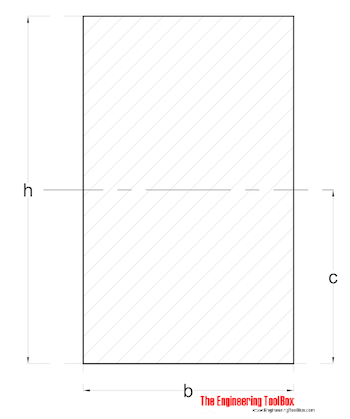Radius of Gyration for a rectangle with axis in center can be calculated as

rmax = 0.289 h                                (1)

where

rmax = max radius of gyration (strong axis moment of inertia)

#### Rectangle - with excentric axis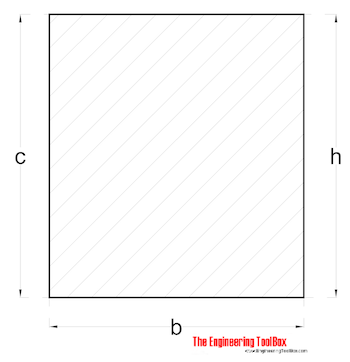Radius of Gyration for a rectangle with excentric axis can be calculated as

r = 0.577 h                                (2)

#### Rectangle - with tilted axis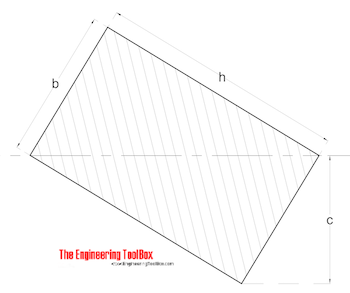Radius of Gyration for a rectangle with tilted axis can be calculated as

r = b h / (6 (b2 + h2))1/2                                (3)

#### Rectangle - with tilted axis II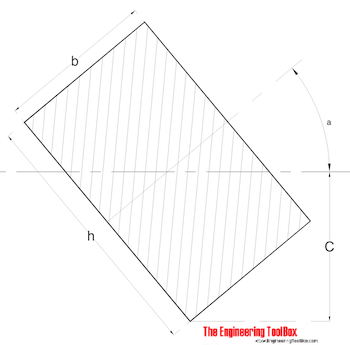Radius of Gyration for a rectangle with tilted axis can be calculated as

r = (((h2 + cos2a) + (b2 sin2a)) / 12)1/2                                (4)

#### Hollow Square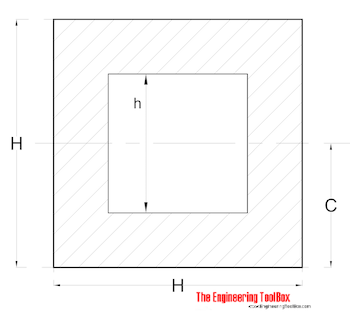Radius of Gyration for a hollow square can be calculated as

r = ((H2 + h2) / 12)1/2                                (5)

#### Hollow Square - with tilted axis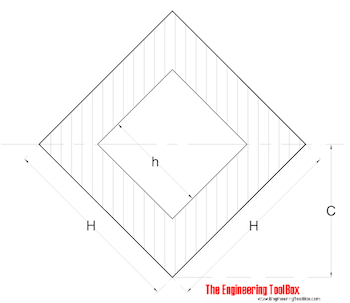Radius of Gyration for a hollow square with tilted axis can be calculated as

r = ((H2 + h2) / 12)1/2                                (6)

#### Equilateral Triangle with excentric axis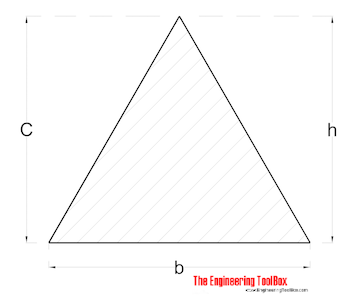Radius of Gyration for a equilateral triangle can be calculated as

r = h / (18)1/2                                (7)

#### Triangle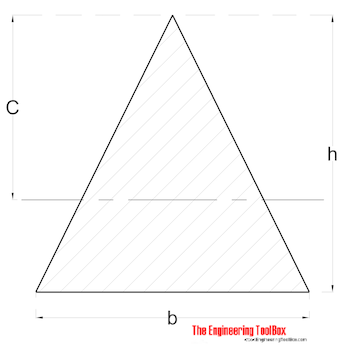Radius of Gyration for a equilateral triangle can be calculated as

r = h / (6)1/2                                (8)

## Related Topics

• ### Beams and Columns

Deflection and stress, moment of inertia, section modulus and technical information of beams and columns.
• ### Mechanics

Forces, acceleration, displacement, vectors, motion, momentum, energy of objects and more.

## Related Documents

• ### Area Moment of Inertia - Typical Cross Sections I

Typical cross sections and their Area Moment of Inertia.
• ### Area Moment of Inertia Converter

Convert between Area Moment of Inertia units.
• ### Euler's Column Formula

Calculate buckling of columns.
• ### Mass Moment of Inertia

The Mass Moment of Inertia vs. mass of object, it's shape and relative point of rotation - the Radius of Gyration.
• ### Pipe Formulas

Pipe and Tube Equations - moment of inertia, section modulus, traverse metal area, external pipe surface and traverse internal area - imperial units
• ### Square Hollow Structural Sections - HSS

Weight, cross sectional area, moments of inertia - Imperial units
• ### Structural Lumber - Section Sizes

Basic size, area, moments of inertia and section modulus for timber - metric units.

## Engineering ToolBox - SketchUp Extension - Online 3D modeling!

Add standard and customized parametric components - like flange beams, lumbers, piping, stairs and more - to your Sketchup model with the Engineering ToolBox - SketchUp Extension - enabled for use with older versions of the amazing SketchUp Make and the newer "up to date" SketchUp Pro . Add the Engineering ToolBox extension to your SketchUp Make/Pro from the Extension Warehouse !

We don't collect information from our users. More about

## Citation

• The Engineering ToolBox (2008). Radius of Gyration in Structural Engineering. [online] Available at: https://www.engineeringtoolbox.com/radius-gyration-structural-engineering-d_1331.html [Accessed Day Month Year].

Modify the access date according your visit.

9.19.12